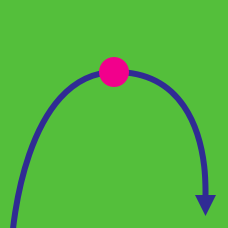Calculus

# Extrema: Level 4 Challenges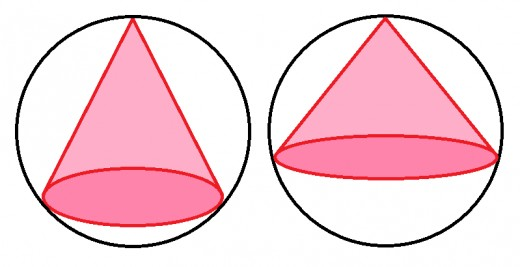If the largest possible volume of a cone inscribed in a sphere of unit volume can be represented as $\frac{a}{b}$, where $a$ and $b$ are coprime natural numbers, what is the value of $a+b$?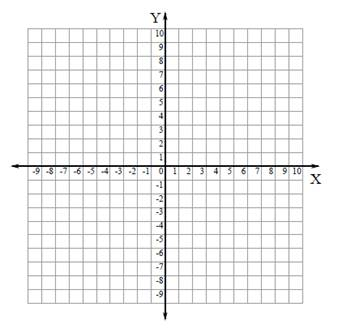On a Chocolate Day , A Mathematician(Romeo) starts from his house which is located at point P(-3,4) and wants reach at His Girlfriend(Juliet) House which is located at point Q(0,1). But instantly he think that he Should buy chocolates for his Girlfriend(Juliet) to impress her. So He went to shop which is located at coordinate X-axis at point R($\alpha$.,0) .But he keep in mind that he should reach to his Girlfriend's House by travelling minimum distance otherwise She will be angry. As he is an Mathematician so he Chose a particular shop so that he reach His Girlfriend's House by travelling minimum Distance.Then find the value of $\alpha$. so that he will impress his Girlfriend.

Let $\alpha$=$\frac { a }{ b }$. Where 'a' and 'b' are coprime integers.

Then find the value of $\quad |a\times b|$.

DETAILS AND ASSUMPTIONS

$\bullet$ Assume that Mathematician is moving in Co-ordinate X-Y plane.

$\bullet$ There are infinite number of Shops at Co-ordinate X-axis.So Mathematician(Romeo) choose Particular Shop To impress his Girlfriend(Juliet).

$\bullet$ Treat all object as point object.

$\bullet$ May be Fermat's Principle of Light waves Helpful Here .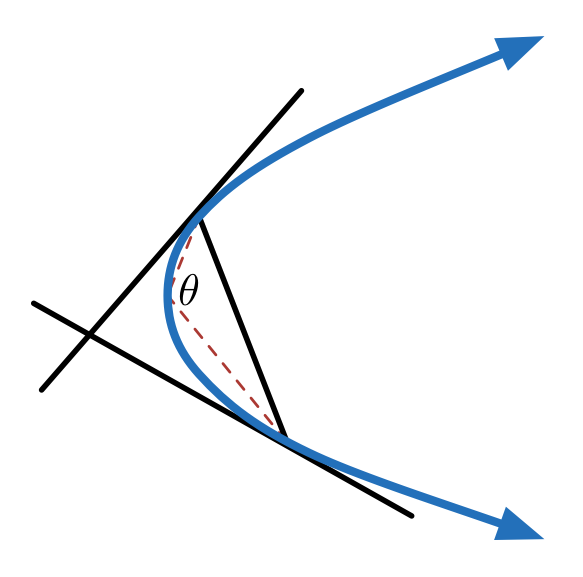Two points are chosen at a parabola, such that tangents at these points are perpendicular to each other . Let the angle subtended by the chord joining the points at the vertex of the parabola be $\theta$ . Find the value of $\theta_{max} + \theta_{min}$ in degrees.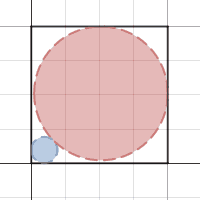Consider a square $ABCD$. Two circles $X$ and $Y$ are drawn such that circle $X$ is tangent to two adjacent sides $AB$ and $AD$, and circle $Y$ is tangent to the other two adjacent sides $CB$ and $CD,$ and circles $X$ and $Y$ are tangent to each other.

As the radius of circle $X$ increases, at what radius of circle $X$ does the sum of the areas of circle $X$ and $Y$ change the slowest?

Express that radius as a fraction of the side of the square $ABCD$, and round to the nearest hundredth.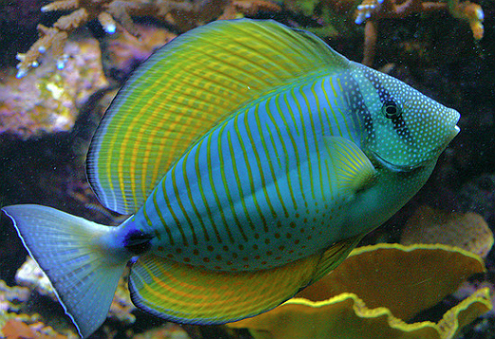Find the minimum possible value of $|a|$ such that the function below is continuous along the interval $[b,\infty)$ and there is no value of $x such that $y$ is a real number.

$16y^2=a+15 x-9 x^2+x^3$

Note: $b$ is a constant of real integral value.

×